### 一、引言

#### 1.2 跨域推荐的细节

• 用户与物品之间没有都没有交集；
• 两个域的用户有部分交集，但是物品没有交集。这种情况可以理解成鬼畜用户和舞蹈用户会有部分交集，这部分用户他们既访问了鬼畜区的视频又去舞蹈区看了小姐姐；
• 这个场景的重合度就比较高了，不论是用户还是物品都有一定程度上的重叠，这在b站上也是很常见的，比如自制区的视频同时也是数码区的视频。

### 二、模型设计

#### 2.1 数据集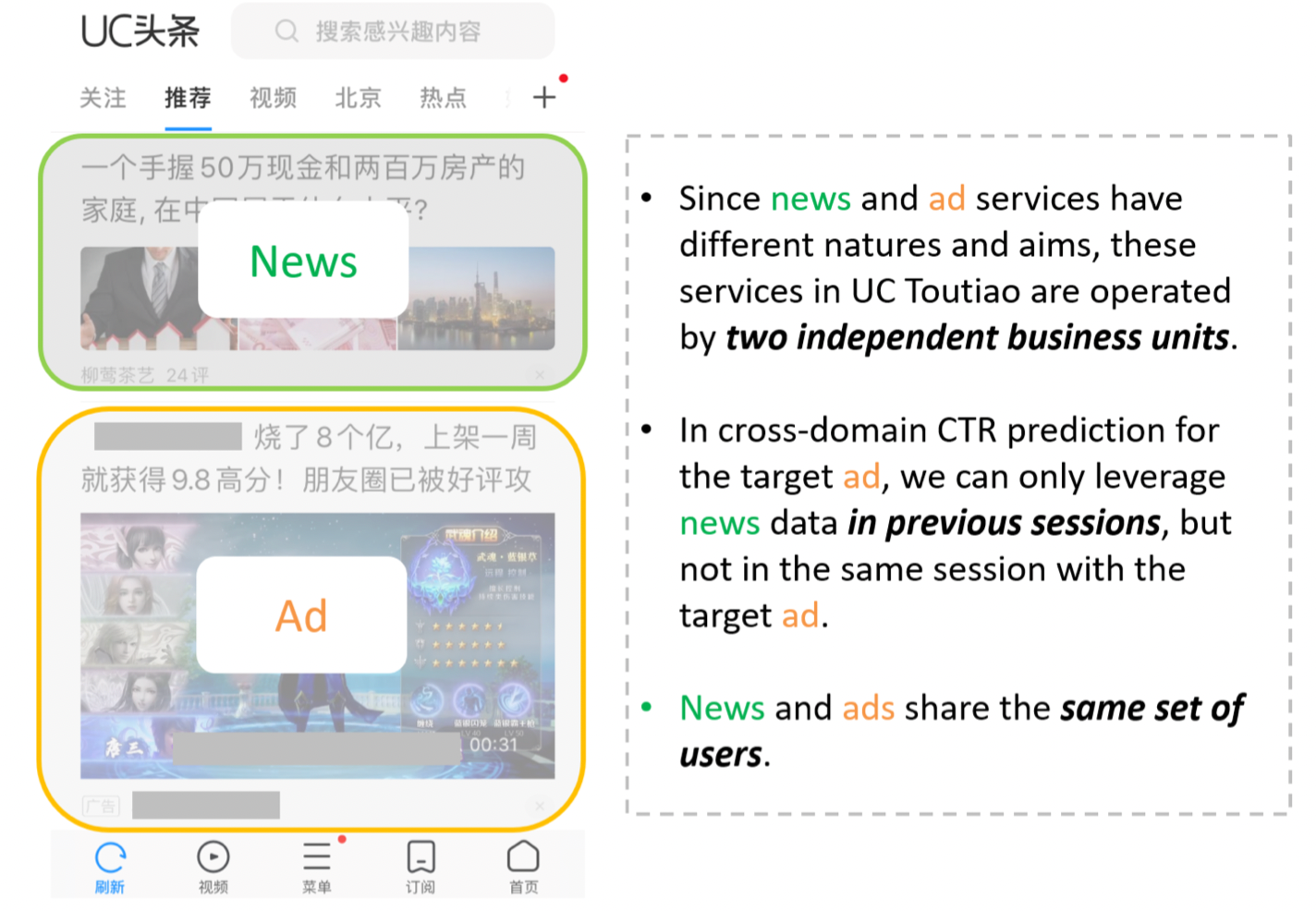#### 2.2 结构设计

• 跨域长期兴趣（Long-term interest across domains）：用户的profile feature能反映出他长期的、固有的兴趣。通过跨域数据（用户交互过的所有的新闻和广告记录）可以学习出一个包含更多语义信息和可信度更高的user embedding。简单来说，就是通过用户的基本信息建模用户的内在长期兴趣。

• 源域短期兴趣（Short-term interest from the source domain）：对于每个待预估的广告，都会有一个源域的短期用户兴趣与之关联，尽管广告和新闻的内容可能是完全不同，但其中很可能会存在一个确定的相关性比，如看了娱乐新闻后的用户可能会去点击游戏广告。基于这种相关性，我们能把源域的有用信息迁移到目标域来。简单来说就是对用户在源域的短期行为进行建模。

• 目标域短期兴趣（Short-term interest from the target domain）：这个不言而喻了，就不多阐述了，简单来说就是对用户在目标域的短期行为进行建模。

• 不是所有交互过的新闻都和目标广告有关系
• 同样，也不是所有交互过的广告都和目标广告有关系
• 模型必须能把信息从源域迁移到目标域
• 对于每个目标广告，三种用户兴趣的重要性是不一样的
• 用户兴趣向量的维度可能不一样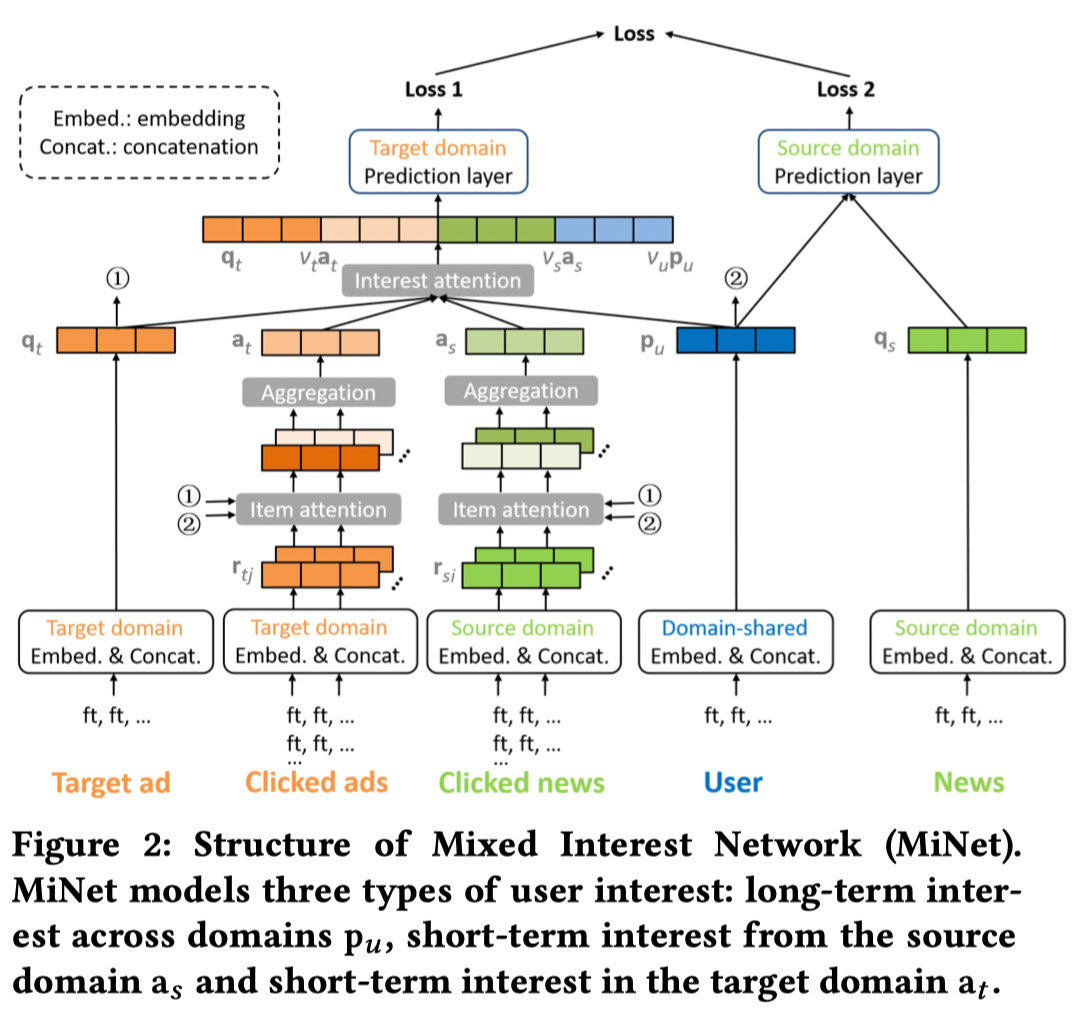#### 2.3.1 Embedding层

type feature
User information user ID, city, age…
Ad information creative ID, campaign ID, title…
News information creative ID, campaign ID, title…#### 2.3.2 跨域长期建模

$$P_u=[e_{u123}||e_{BJ}||e_{male}||e_{ios}]$$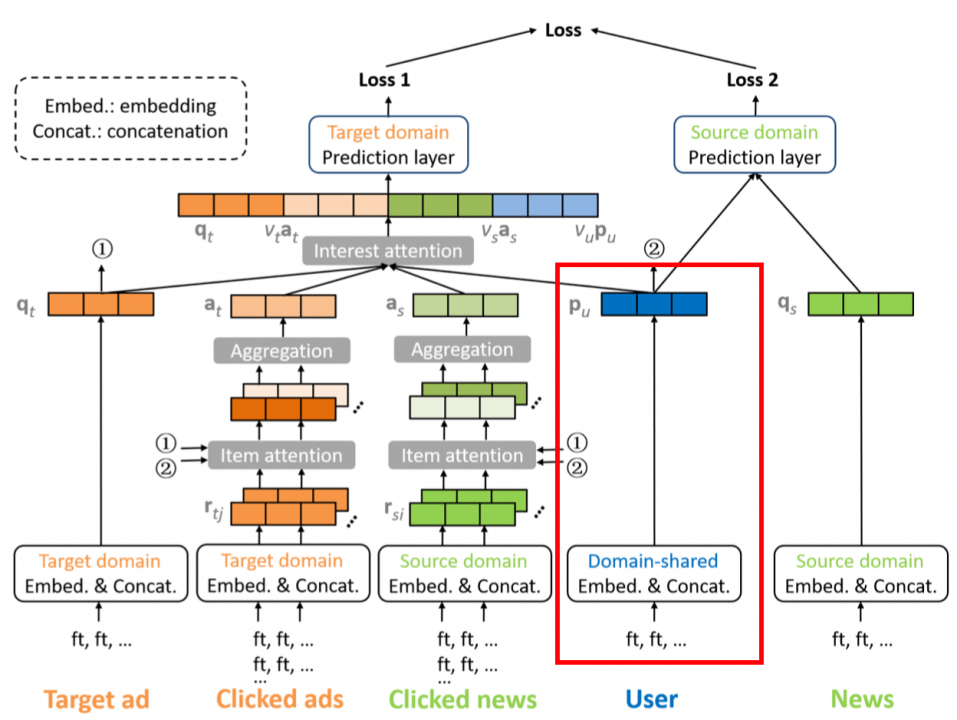#### 2.3.3 源域短期兴趣建模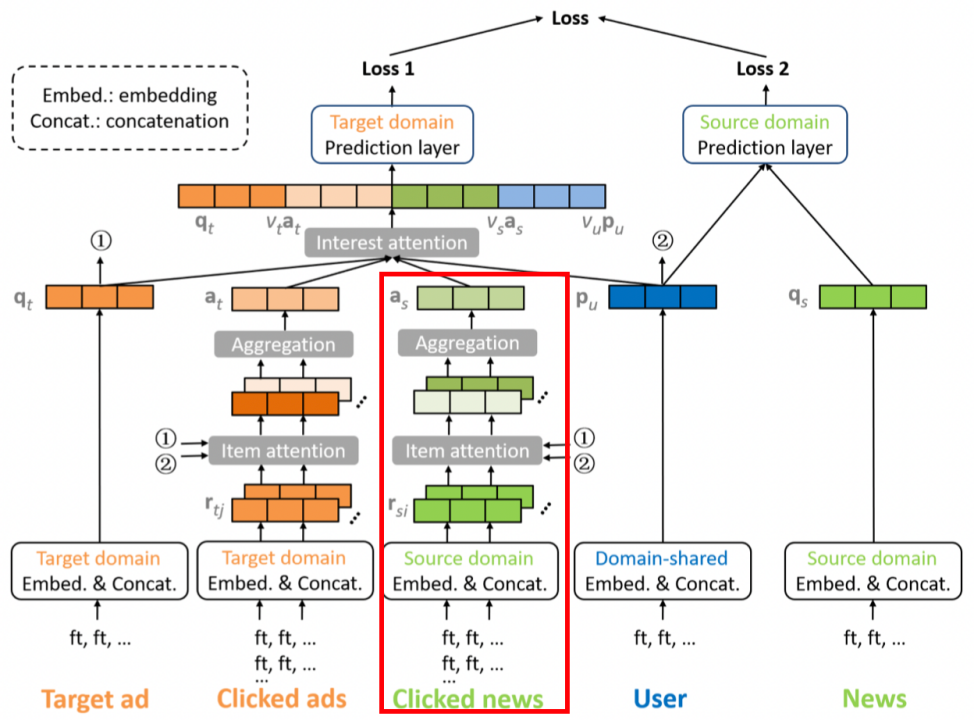##### 2.3.3.1 Item attention

$$\widetilde{a_i}=h_s^TReLU(W_s[r_{si}||q_t||p_u||Mr_{si}\odot{q_t}])$$

• $r_{si}$ 是源域被点击新闻embedding并拼接后的特征向量；
• $q_t$ 是目标域目标广告embedding并拼接后的特征向量；
• $p_u$ 是用户长期兴趣向量；
• $Mr_{si}\odot{q_t}$ 中，$M$ 是待学习的矩阵，其将 $r_{si}$ 映射到目标域空间中与$q_t$ 作元素积；
##### 2.3.3.2 Aggregation

$$a_i =\frac{exp(\widetilde{a_i})}{\sum{exp(\widetilde{a_i})}}$$

$$a_s=\sum{a_ir_{si}}$$

#### 2.3.4 目标域短期兴趣建模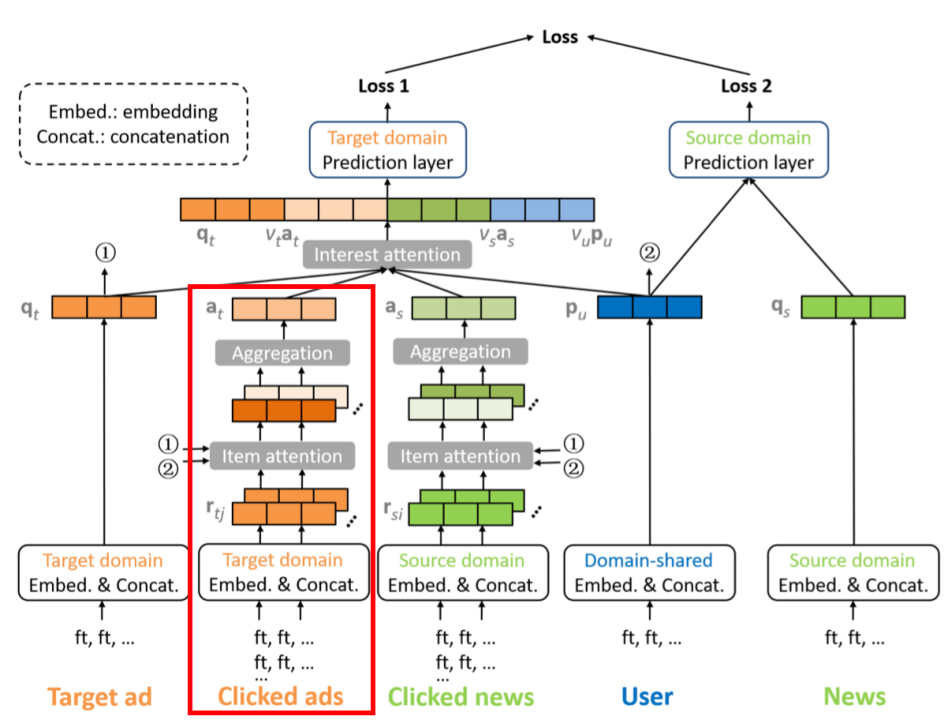##### 2.3.4.1 Item attention

$$\widetilde{\beta_j}=h_t^TReLU(W_t[r_{tj}||q_t||p_u||r_{tj}\odot{q_t}])$$

##### 2.3.4.2 Aggregation

$$\beta_j =\frac{exp(\widetilde{\beta_j})}{\sum{exp(\widetilde{\beta_j})}}$$

$$a_t=\sum{\beta_jr_{tj}}$$

#### 2.3.5 Interest Attention层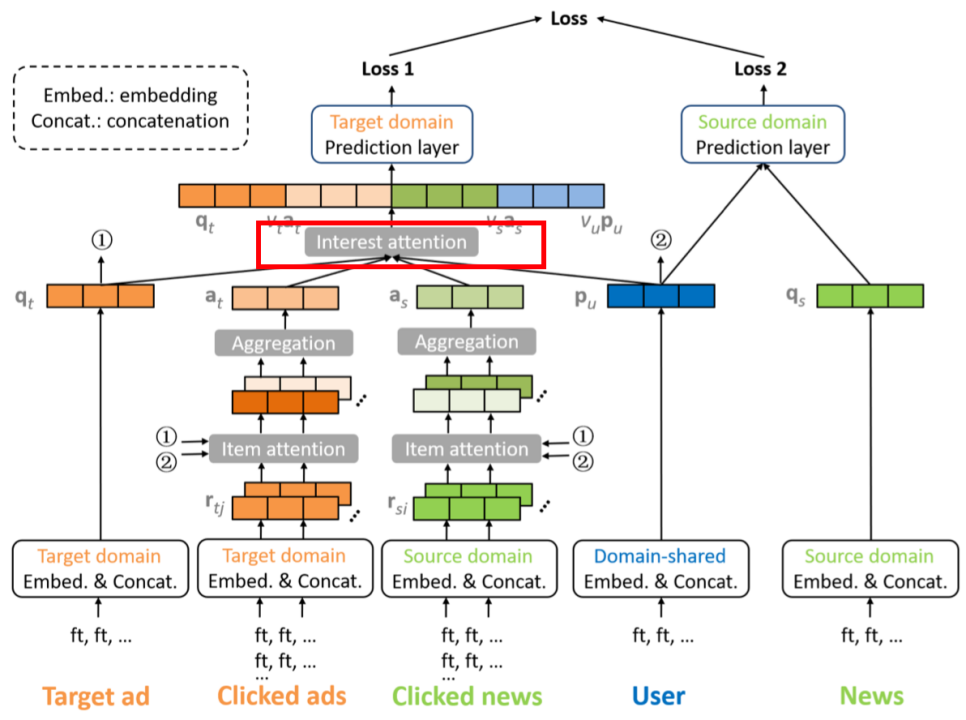$$v_u=exp(g^T_uReLU(V_u[q_t||p_u||a_s||a_t])+b_u)$$

$$v_s=exp(g^T_sReLU(V_s[q_t||p_u||a_s||a_t])+b_s)$$

$$v_t=exp(g^T_tReLU(V_t[q_t||p_u||a_s||a_t])+b_t)$$

#### 2.3.6 Prediction层和Loss函数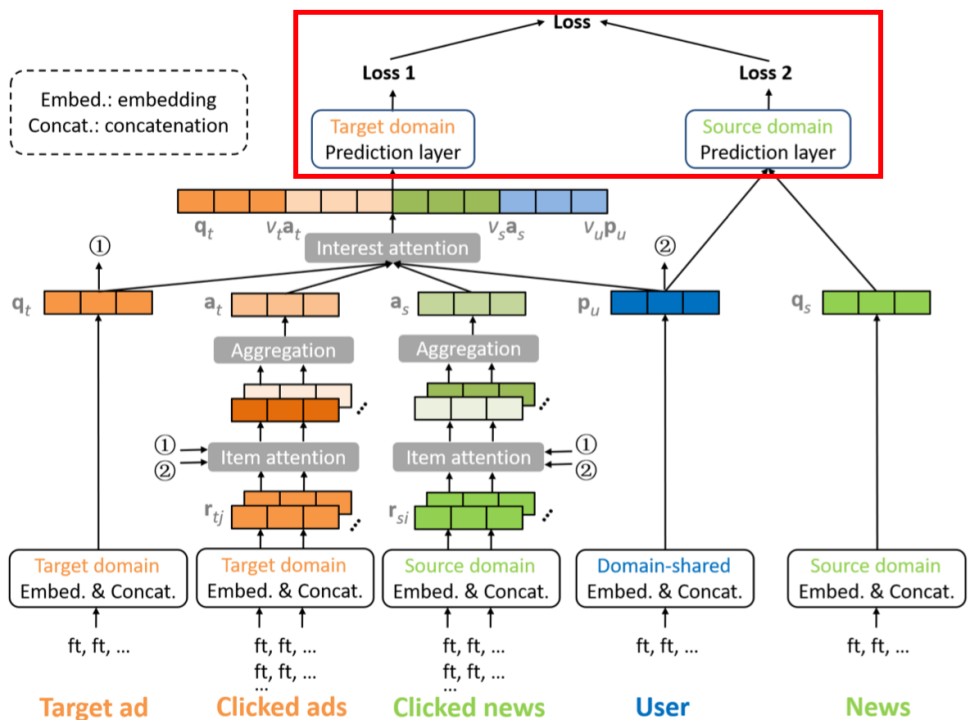$$loss=loss_t+\gamma loss_s$$

### 三、跨域推荐优缺点

• 首先，它可以用来解决一部分冷启动的问题。目标域的新用户很可能是源域的旧用户，那么将源域的信息拿过来辅助提升推荐的效果，能一定程度上解决冷启动；
• 第二点就是提升目标域的推荐效果，这个也是跨域推荐的主要目的；
• 第三个优势是多样性。因为跨域推荐同时参考了多个域的特征，自然而然会对推荐结果的多样性进行一定的优化。最终，它还会反作用于源域，能够实现源域的推荐与目标的域推荐效果的共同提升。

• 跨域推荐还需要考虑一定的权衡，因为跨域必然会导致数据的稀疏，处理不当可能会有反作用。

### 四、参考链接微信支付宝